# How to rewrite this constraint function subject to DCP rules?

Hi there,

I’m trying to solve the following optimization problem:where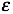and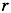are both n-by-1 vectors, I wrote in matlab the following:

``````cvx_begin

variable X(2*n,1) nonnegative
minimize [ones(1,n), zeros(1,n)] * X;
subject to
log(1 + c * pow_p([eye(n),zeros(n)]*X,-2)) + log(1+power(1/4,[zeros(n),eye(n)]*X)) <= d;
[zeros(1,n),ones(1,n)] * X <= R;

cvx_end
``````

I’ve managed to write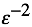and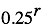according to DCP rules using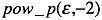and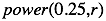respectively, but there’s still the problem of log{ (convex) } of the first term in the first constraint function. But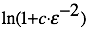is indeed convex.

So my question is how to rewrite the first constraint function that obeys the DCP rules?

Thank you.

Unfortunately, I doubt it is possible. CVX will likely not be able to solve this problem. I will take it at your word that this function is convex, nevertheless, CVX does not attempt to support every convex problem, as discussed in the FAQ.